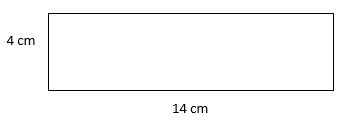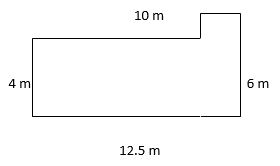# Imperial Units and Perimeter of Straight-line Shapes

Imperial Units

While we have made a mathematically much-needed move to a metric system, there are many of us of a certain age who will forever see things in terms of miles, gallons, feet, pounds and so on. While the curriculum requires children to be aware of these different units, it does not require them to carry out complex calculations using them. Most work that they will do simply sees them convert between an imperial and metric unit.

The basic need-to-know information is this:

 Measure: a mile is about 1.6 km (5 miles = 8 km is a useful aid) a yard is about 90 cm a foot is about 30cm (One of those 30cm rulers is great to show for this) an inch is about 2.5 cm

12 inches make a foot, 3 feet make a yard, 1760 yards make a mile

 Mass: 1 kg is about 2.2 lbs (pounds) an ounce is about 30 g

16 ounces make a pound, 14 pounds make a stone

 Volume: a pint is about 0.6 litres a gallon is about 4.5 litres

There are 8 pints in a gallon and 20 fluid ounces in a pint.

The likelihood is that the papers will provide information like this or a more precise measurement if they want an accurate conversion. However, some of this knowledge will be expected, such as the average person being between 5 and 6 feet tall, and basic conversion from there to metres.

It is also possible to see a conversion between degrees Fahrenheit and Centigrade; this is not easy to explain so is much more straightforward to point out that 0°C = 32°F and, as a guideline, 20°C = 68°F.

Perimeter of Straight-line Shapes

The perimeter of a shape is the distance around the outer edges. If a shape has the length of each and every line on it, simply add the figures together and give the answer. Do not write it as m2 or cm2; it is simply a total of metres or centimetres and does not need the 'squared'.

If you are asked to find the perimeter of a square, it is four times the length of one side. If you are given any other regular polygon with one known side, you follow the same process - multiply the length of one side by the number of sides. A regular hexagon with one side of 5cm will have a perimeter of (5 x 6) 30cm.

When asked to give the perimeter of a rectangle, it is usual to have the length of two sides provided; you should add the two together and multiply by two to give the answer - if it helps, write on the measurements on a diagram and add the figures. A lot of children prefer this visualisation.The perimeter will be ((4 + 14) x 2) = 36 cm.

Most questions that are given at this age level will leave some line lengths without labels. Your child is expected to be able to work out the missing lengths and calculate accordingly.

Look at this example:The unwary candidate (and I've taught many!) will simply add the given lengths. They are missing the fact that the shape has six sides - the two little ones in the top right corner must be worked out too.

The vertical one is 2m as if it is added to the 4m on the left it should make 6m, the length of the rightmost line. The horizontal line is (12.5 - 10) = 2.5 m.

Now we know the length of all six sides, simply add them to get the perimeter.

10 + 4 + 12.5 + 6 + 2.5 + 2 = 37 m

However, there is a quicker way for all shapes that look like this. If you think about the total length (12.5 m) and the total height (6 m) then double them, you actually get to the answer, just as you would with a complete rectangle! It's a handy trick and works for all rectangles which have a 'corner removed'.